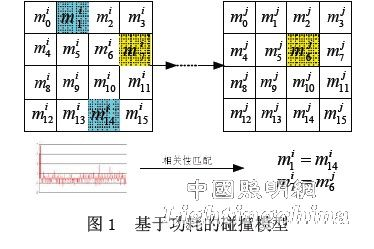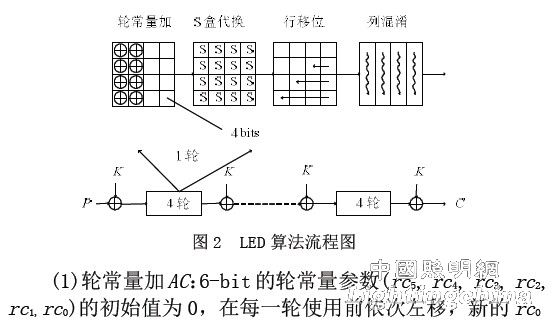# LED algebra bypass attack based on collision model

introduction

As an important part of modern cryptography, block cipher is of great significance for ensuring information security. With the development of information technology and electronic components, cryptographic devices have gradually become lighter. How to implement cryptographic algorithms on lightweight cryptographic devices such as RFID tags has become a new hotspot in block cipher research. Lightweight block ciphers cannot be ignored due to their particularity in resource-constrained application environments. This article takes the LED light block code  as the research object.

Algebraic bypass attacks  combine algebraic attacks  with bypass attacks , using algebraic attacks to establish a cryptographic algorithm for plaintext and key algebraic equations, and then by means of bypass attacks The leaked information obtained is substituted into the equations to help solve the problem. This combination overcomes the high complexity of the algebraic attack to solve the equations, and makes up for the shortage of sample size, low utilization of bypass information, low number of analysis rounds, poor applicability, etc. The inevitable result of the development of bypass attacks. Algebraic bypass attacks can recover keys in the case of unknown plaintext, and sometimes only one sample can recover the keys. For some password implementations that incorporate defenses, attacks can be successfully implemented, giving block ciphers, especially light block ciphers. Caused a serious security threat. How to perform efficient key recovery based on algebraic bypass attacks has important theoretical and practical significance.

Power consumption collision information is used as a kind of bypass information for cryptanalysis. It was first used by Wiemers  and others in 2001 to attack hardware-implemented ECC. Schramm first used collision model-based power attack in 2003. Applied to block ciphers .

In this paper, LED light block code is used as the attack object. Based on the collision model, the power consumption leakage during the execution of the LED algorithm on the microcontroller is collected. The Pearson correlation coefficient  method is used to match and convert the power consumption value of the encrypted intermediate state. For the algebraic equations, the CryptoMinisat satisfiability parser  is used to solve the key. The results of the attack experiments show that the LED is vulnerable to algebraic bypass attacks. It is known that the collision information output by the two rounds of S-box under plaintext conditions can recover all the initial keys.

The organization of this paper is as follows: Section 1 mainly gives the collision model and algebraic bypass attack principle, Section 2 builds the LED algebraic equations, Section 3 is based on the power consumption collision capture and collision representation, and Section 4 gives the experiment. As a result, the final section 5 summarizes the article.

1 Algebraic bypass attack principle based on collision model

1.1 Collision model

Collision meaning means that two or more different things meet at a certain point, and the extension is of some nature. In a block cipher algebra bypass attack, if the value of any two or more intermediate states is the same when the algorithm is running, a collision occurs. Converting the collision information into an equation can expand the algebraic equations of the algorithm and reduce the complexity of solving the key.

Collisions of intermediate values â€‹â€‹in actual attacks can be manifested by leakage of bypass information, such as power consumption. The power consumption of the cryptographic device is not only related to the cryptographic device, but also closely related to the processed data. Given the power consumption curve of a cryptographic algorithm at runtime, correlate the power trajectories corresponding to two intermediate values â€‹â€‹(LEDs are nibbles) output from multiple rounds of S-boxes, and calculate the correlation between power trajectories. The coefficient of significance, thereby inferring whether the two intermediate values â€‹â€‹collide.

Figure 1 is a collision model, showing the process of collision capture and utilization of any two intermediate states in the algorithm operation, where m is the state matrix, and i and j are the number of rounds. Taking the S-box output of two adjacent rounds as an example, correlation matching is performed on the corresponding part of the power consumption curve. After the matching is successful, the collision information can be acquired, and the captured collision information can be converted into an algebraic equation.1.2 Attack principle

Algebraic bypass attacks can generally be divided into three steps: cryptographic algorithm equations representation, bypass leakage acquisition and utilization, and algebraic equations.

(1) Cryptographic algorithm Equations representation: The cryptographic algorithm is represented as an algebraic equations for plaintext, keys, intermediate variables, ciphertext. In the construction process of the algebraic equations of the LED algorithm, the most critical part is how to construct the algebraic equations of the S-box of the nonlinear component.

(2) Bypass Leakage Collection and Utilization: Given an algebraic equation system of a cryptographic algorithm, key recovery is equivalent to solving algebraic equations. Directly solving algebraic equations is an NP problem, so it is necessary to use the bypass leakage information to reduce the complexity of the equations. The attacker can select a bypass leakage model M according to the password implementation platform and its own measurement capability, and then collect the bypass leakage information L, convert L into the encrypted intermediate state D according to the leakage model M, and finally use D for additional The algebraic equations are represented. In this paper, the LED algebra bypass attack is based on the collision model, and the collected bypass leakage information is power consumption information.

(3) Solving algebraic equations: The previously established cryptographic algorithm equations are connected with the bypass leakage algebraic equations, and then the equations are solved. At present, typical algebraic equation solving mainly includes methods based on satisfiability problem solving, linearization and GrÅbner basis. In this paper, the algebraic equations are solved based on the satisfiability (SAT) problem solving method. First, the equations are transformed into SAT problems, and then the SAT parser is used to solve the key. This paper uses the CryptoMinisat parser as a tool for solving algebraic equations.

2 LED algebra equations construction

2.1 Algorithm Analysis

The LED Lightweight Block cipher uses an SPN architecture that requires only 966 gates and is the least of the same type of block cipher. Due to its structural characteristics, many AES-like theorems can be derived, and the ability to resist differential, linear, and algebraic attacks is extremely strong. However, the ability to resist algebraic bypass attacks is not considered in the algorithm design, and the algebraic bypass attack is used to evaluate the security. And protection has important significance.

The LED algorithm has a packet length of 64 bits and supports a key length of 64/128 bit, and the number of encryption rounds is 32 rounds. In this paper, the LED block cipher refers to the algorithm of the 64 bit key. The round key is added before the first round of the algorithm, and then every 4 rounds, that is, there are 9 round key addition operations in all rounds. In order to improve the encryption speed and reduce the hardware implementation scale, a keyless generation strategy is adopted, and the round key is the initial key. The state matrix of the algorithm is a 4*4 matrix on GF(24) with 4 bits per element. There are 4 steps in each round with round constant addition, S box, line shift and column confusion. The specific parameters are shown in the literature , and Figure 2 is a flow chart of the algorithm.